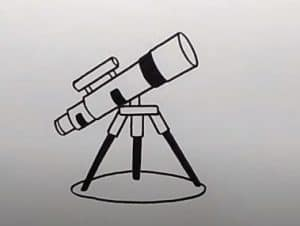# How to Draw a Telescope easy for beginners

How to Draw a Telescope easy with this how-to video and step-by-step drawing instructions. Easy drawing tutorial for beginners and kids.## You can refer to the simple step-by-step drawing guide below

### Step 1

Start by sketching out the telescope objective. Draw a pair of lines, slightly diverging from each other. Connect them with curves at each end. From the narrow end, extend another pair of straight lines and connect them with a curve. At the wide end, details the lens using two curves to surround an oval part.

### Step 2

Draw optical tubes. Extend a pair of curves from the bottom of the lens. The lines should converge gently. Connect them at the end with a curve and wrap this end of the tube with an additional curve.

### Step 3

Draw the mount, erase as needed. Use straight and curved lines to outline a “Y” shape around the telescope lens. Draw curves and straight down to the “Y” shape to give the stand a three-dimensional shape.

### Step 4

Draw scope finder. Stretch a pair of short lines from the top of the telescope. Connect them at the ends with a straight line. Draw another line above the previous one and connect them with a pair of parallel curves. Extend the lines on each side of this shape and connect them at the end with curves. Wrap the lens with curves. Use two curves to surround the shape of the lens at one end.

### Step 5

Continue pipes by stretching a pair of straight lines. Then, surround a cylinder with two lines and two curves. Draw a “C” shaped line over the shape. This is the focus knob. Finish wrapping the pipe with a curve. Then stretch two more lines and connect them with a curve.

### Step 6

Draw the diagonal of the star. Cover a trapezoid at the end of the tube, partially obscured by it. Draw a line through it, giving it a three-dimensional shape. Extend the two lines above it and connect them with a curve; then, expand two more and connect them. Draw a curve around the end of this shape. This forms the eyepiece of the telescope.

### Step 7

Continue drawing the mount. Surround a small cylinder using two curves and two lines, erasing as needed. Draw a “C” shaped line through it. This is a good height control knob. Then surround a pentagon, partially obscured by the knob. Draw a line along its length to give it a three-dimensional shape.

### Step 8

Draw the legs of the tripod. For each leg, stretch a pair of lines and connect them with a curve; say again. Use a curve to surround the leg of the leg.

### Step 9

Draw the other tripod.